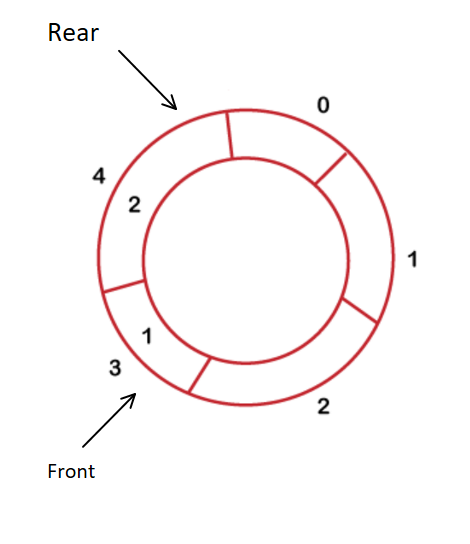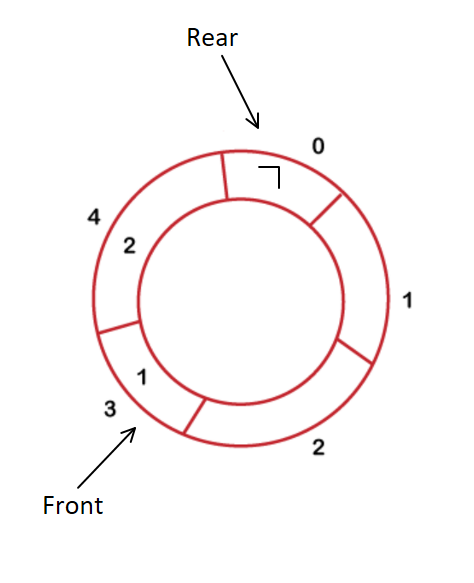# Advantages of Circular Queue over Linear Queue

## Introduction

In this article, we’ll see the advantages of a Circular Queue over Linear Queue. Although each data structure has its advantages and disadvantages, each is used at specific places. We will first see what linear and circular queues are, then compare them, and lastly, the advantages of a circular queue over a linear queue.

Let’s move on to what are circular and linear queues without any ado.

## What is a Linear Queue?

A linear queue is generally referred to as Queue. A linear queue is an abstract linear data type with a predefined capacity.

Queue follows the “First in First Out” (FIFO) principle; that is, the element which comes first will be removed first, just as in an actual queue you would see at a supermarket. A queue is open from both ends, one end is for inserting, and the other is to remove the data. There are two main functionalities in a linear queue: Enqueue and Dequeue. Enqueue helps insert the data from one end, whereas Dequeue helps remove the data from the Queue.

## What is a Circular Queue?

A circular Queue is similar to a linear Queue, and it’s also based on the First In First Out(FIFO) principle. The only thing that differentiates it from a linear queue is that the rear and the front ends are connected in this Queue. There is no end in this Queue. This specialty gives rise to a lot of benefits.

• When enqueue is performed on a queue/circular queue, an element is added to the index where the rear end is pointing.
• While, when dequeue is performed on a queue/circular queue, an element is removed from the index where the front is pointing to.

## Comparison between Linear and Circular Queues

In a linear Queue, the front starts pointing to the next element after removal, and the index where the front was pointing earlier remains empty. Now, if the Queue size is five and the rear-end points to the last element, we can’t add more elements to this Queue.

Whereas in a circular Queue, after removal, the index where the front was pointing earlier becomes empty, but elements can be inserted into it again when the rear endpoints to this index. Since it is circular, there is no end. If the size is, let’s say, 5, the front is pointing to index 0, and the rear is pointing to index 4. If we dequeue, the index 0 becomes empty. If we want, we can add more elements to this Queue as the rear can move to index 0 here.In the above example picture, three elements have been dequeued. Rear points to index four and front points to index three now. Then, if we want to add element 7, we can add it to the index 0 as the rear end moves circularly here. This is the advantage of a circular Queue over a linear Queue.

## Advantages of the circular queue over linear queue

• Flexible in insertion-deletion: In a circular queue, elements can be added easily if the Queue isn’t full. But in the linear Queue, elements can’t be added furthermore, once the rear-end points to the last index.

• Memory efficiency: Circular Queue is memory more efficient than a linear Queue as we can add elements until complete. Thus, no space is left over. While in a linear queue, once the Queue is full, if we start to dequeue, the front indexes become vacant, and then they can never be filled. Thus, there is a wastage of space.

If you are looking for a one-stop destination to master Data Structures like Stacks and Queues, watch the below video for the complete roadmap.

### What are queues in data structure?

A queue is a data structure that follows the property of first in, first out. It is the interconnection of nodes containing all kinds of data.

### What are the types of Queues in the data structure?

There are four kinds of queues available – simple Queue, Circular Queue, priority queue, and double-ended Queue.

### Write the application of Queues.

We use a queue in places where data has to be stored but not processed immediately. Some examples include – CPU scheduling, printer queue, phone calling system, etc.

### Why is a circular Queue used?

Circular Queues offers a quick and clean way to store FIFO data with a maximum size. It provides efficient memory here. That’s why it’s convenient to use.

## Conclusion

This article discussed the differences and advantages between circular Queues and linear Queues in this article. You can look up more information on the queue data structure. Some problems based on linear Queue are: reversing a queuereversing the first k elements of a queue, and implementation of Queue. Also, look into more operations and problems related to queues. You can try these out to gain more confidence on this topic. These questions are asked during various coding contests as well as placement tests.

To practice more such problems on Data Structures and AlgorithmsCodestudio is a one-stop destination. This platform will help you acquire effective coding techniques and overview student interview experiences and interview bundle in various product-based companies.

Happy Coding!